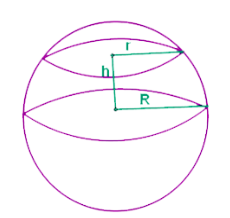# Spherical Segment Formula

If we cut a basketball with a pair of two knives parallely, then the solid that is defined by the cutting is the spherical segment. You can say that the spherical cap has been truncated, and so it can be called as a spherical frustum.Those spherical segment is called spherical zone, where we exclude the bases.The formula that we use here are volume and surface area:

$\large A=2\pi Rh$

$\large V=\frac{\pi h}{6}(3r_{1}^{2}+3r_{2}^{2}+h^{2})$

### Solved Example

Question: What will be the volume of a segment of a sphere,the radius of the base being 9.2 cms, the radius of sphere 11 cms and height is 7 cms ?

Solution:

Using the formula:

$$\begin{array}{l}V=\frac{\pi h}{6}(3r_{1}^{2}+3r_{2}^{2}+h^{2})\end{array}$$
$$\begin{array}{l}V=\frac{\pi \times 7}{6}(3\times 9.2^{2}+3\times 11^{2}+7^{2})\end{array}$$
$$\begin{array}{l}= 3.66 \times 665.92\end{array}$$

= 2441.70